# MINS/BDS组合导航系统设计与实现Design and Implementation of MINS/BDS Integrated Navigation System

DOI: 10.12677/JSTA.2019.73010, PDF, HTML, XML, 下载: 404  浏览: 923

Abstract: IIn order to meet the requirement of low cost and light weight navigation system for Military Un-manned detection vehicle, the design of MINS/BDS integrated navigation system is completed by using the MEMS inertial navigation system (MINS) and three-antenna Beidou receiver (BDS). The integrated navigation calculation module is designed based on ARM + FLASH, which realizes the functions of multi-sensor communication, attitude calculation, orientation and positioning, and human-computer interaction with the host computer. Computing module, power module, filter module, MIMU and Beidou data receiving module are integrated into the embedded MIMU/Beidou integrated navigation system. The completed system has a diameter of 78 mm, a height of 60 mm and a quality of no more than 600 g. The fixed-point dynamic attitude experiment of the integrated system shows that the output frequency of the integrated navigation system is 200 Hz, the fixed-point position measurement accuracy is less than 1 m, the attitude angle of the dynamic at-titude measurement accuracy is less than 0.1 degree and the heading angle is less than 0.5 degree.

1. 引言

2. 组合导航系统方案

MIMU/BDS组合导航系统包括4个部分，其中嵌入式电源模块为MIMU、组合导航计算模块和BDS接收机模块提供电源，MIMU和BDS接收机模块分别通过标准RS-422和RS-232与计算模块进行通信，组合导航计算模块接收到IMU和BDS的测量信息，采用基于卡尔曼滤波的观测控制器方式进行初始对准，之后采用松组合方式进行导航解算，并通过标准RS-422串口和上位机监测软件进行通信。上述4个部分集合成一体，系统框图如图1所示。

3. 算法设计

3.1. 卡尔曼滤波器原理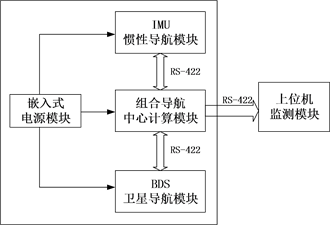Figure 1. Block diagram of embedded integrated navigation system

${X}_{k}={\Phi }_{k,k-1}{Χ}_{k-1}+{\Gamma }_{k,k-1}{W}_{k-1}$ (1)

${Ζ}_{k}={Η}_{k}{Χ}_{k}+{V}_{k}$ (2)

$\left\{\begin{array}{c}Ε\left[{W}_{k}\right]=\text{0}，Ε\left[{W}_{k}{W}_{j}^{T}\right]={Q}_{k}{\delta }_{kj}\\ Ε\left[{V}_{k}\right]=\text{0}，Ε\left[{V}_{k}{V}_{j}^{T}\right]={R}_{k}{\delta }_{kj}\\ Ε\left[{W}_{k}{V}_{k}^{T}\right]=\text{0}\end{array}$ (3)

${\stackrel{^}{Χ}}_{k,k-1}={\Phi }_{k,k-1}{\stackrel{^}{Χ}}_{k-1}$ (4)

${\stackrel{^}{Χ}}_{k}={\stackrel{^}{Χ}}_{k,k-1}+{Κ}_{k}\left[Ζ{}_{k}-{Η}_{k}{Χ}_{k-1}\right]$ (5)

${Ρ}_{k,k-1}={\Phi }_{k,k-1}{Ρ}_{k-1}{\Phi }_{k,k-1}^{T}+{T}_{k,k-1}{Q}_{k-1}{T}_{k,k-1}^{T}$ (6)

${K}_{k}={P}_{k,k-1}{H}_{k}^{T}{\left({H}_{k}{P}_{k-1}{H}_{k}^{T}+{R}_{k}\right)}^{-1}$ (7)

${Ρ}_{k}=\left[Ι-{Κ}_{k}{Η}_{k}\right]{Ρ}_{k,k-1}{\left[Ι-{Κ}_{k}{Η}_{k}\right]}^{T}+{Κ}_{k}{\text{R}}_{k}{Η}_{k}^{T}$ (8)

3.2. 扩展卡尔曼滤波原理

${X}_{k}=f\left({X}_{k-1},k-1\right)+\Gamma \left({X}_{k-1},k-1\right){W}_{k-1}$ (9)

${Z}_{k}=h\left({X}_{k},k\right)+{V}_{k}$ (10)

${X}_{k}\approx f\left(\stackrel{^}{X},k-1\right)+\frac{\partial f\left({\stackrel{^}{X}}_{k-1},k-1\right)}{\partial {\stackrel{^}{X}}_{k-1}^{T}}\cdot \left({X}_{k-1}-{\stackrel{^}{X}}_{k-1}\right)+\Gamma \left({\stackrel{^}{X}}_{k-1},k-1\right){W}_{k-1}$ (11)



$f\left(\stackrel{^}{X},k-1\right)-{\frac{\partial f\left({X}_{k-1},k-1\right)}{\partial {X}_{k-1}^{T}}|}_{{X}_{k-1}={\stackrel{^}{X}}_{k-1}}{\stackrel{^}{X}}_{k-1}\text{=}{\phi }_{k-1}$

${X}_{k}={\Phi }_{k,k-1}+\Gamma \left({\stackrel{^}{X}}_{k-1},k-1\right){W}_{k-1}+{\phi }_{k-1}$ (12)

${Z}_{k}\approx h\left({\stackrel{^}{X}}_{{}_{k|k-1}},k\right)+\frac{\partial h\left({\stackrel{^}{X}}_{{}_{k|k-1}},k\right)}{\partial {\stackrel{^}{X}}_{k|k-1}^{T}}\cdot \left({X}_{k}-{\stackrel{^}{X}}_{{}_{k|k-1}}\right)+{V}_{k}$ (13)

${A}_{k,k-1}={\frac{\partial f\left({X}_{k-1},k-1\right)}{\partial {X}_{k-1}^{T}}|}_{{X}_{k-1}={\stackrel{^}{X}}_{k-1}}$

${H}_{k}={\frac{\partial h\left({X}_{k|k-1},k\right)}{\partial {X}_{k|k-1}^{T}}|}_{{X}_{k|k-1}={\stackrel{^}{X}}_{k|k-1}}$

${Z}_{k}={H}_{k}{X}_{k}+{y}_{k}+{V}_{k}$ (14)

${\stackrel{^}{X}}_{k|k-1}=f\left[{\stackrel{^}{X}}_{k-1},k-1\right]$ (15)

${P}_{k|k-1}={A}_{k|k-1}{P}_{k-1}{A}_{k|k-1}^{T}+{B}_{k-1}{Q}_{k-1}{B}_{k-1}^{T}$ (16)

${\stackrel{^}{X}}_{k}={\stackrel{^}{X}}_{k|k-1}+{K}_{k}\left[{Z}_{k}-h\left({X}_{k|k-1},k\right)\right]$ (17)

${K}_{k}={P}_{k|k-1}{H}_{k}^{T}{\left[{H}_{k}{P}_{k|k-1}{H}_{k}^{T}+{R}_{k}\right]}^{-1}$ (18)

${P}_{k}=\left(I-{K}_{k}{H}_{k}\right){P}_{k|k-1}$ (19)

3.3. MINS/BDS组合方案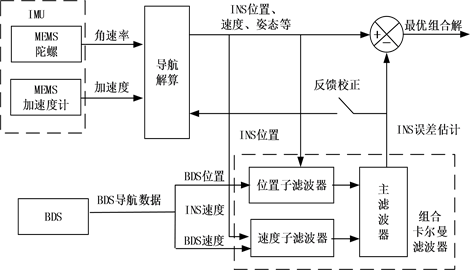Figure 2. Schematic diagram of integrated navigation system

4. 硬件设计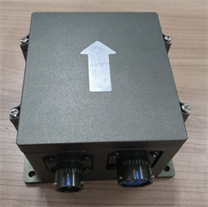Figure 3. Picture of real product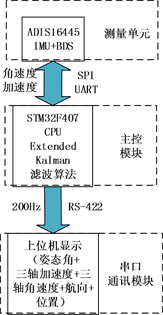Figure 4. Hardware system block diagram

5. 软件设计

ARM芯片开始工作后首先对系统进行初始化，主要工作是关闭看门狗、设置时钟、初始化IO口、初始化UART、SPI、DMA控制器，初始化SDRAM和Flash，记录当前时间等。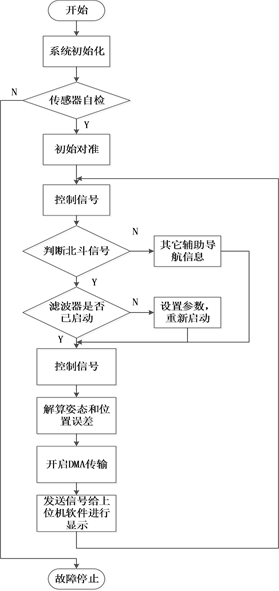Figure 5. Software design flow chart

6. 试验与结果

6.1. 定点位置、速度、姿态航向实验Table 1. Results of fixed point static test

6.2. 定点动姿态航向实验Table 2. Error of fixed point dynamic posture testTable 3. Error of fixed point dynamic heading test

7. 结论

  曾庆化, 刘建业, 彭文明. 我国卫星导航系统相关技术发展分析[J]. 航天控制, 2006, 24(4): 91-96.  张国良, 曾静. 组合导航原理与技术[M]. 西安: 西安交通大学出版社, 2008: 38-42.  谢建东. 无人直升机GPS/INS组合导航系统的设计与仿真[D]: [硕士学位论文]. 南京: 南京航空航天大学, 2005: 25-39.  付梦印, 邓志红, 闫丽萍. Kalman滤波理论及其在导航系统中的应用[M]. 北京: 科学出版社, 2010: 17-33, 160-164.  董绪荣, 张守信, 华仲春. GPS/INS组合导航定位及其应用[M]. 长沙: 国防科技大学出版社, 1998: 215-220.  Yang, Y. (2008) Tight Coupled MEMS INS/GPS Integration with INS Aided Receiver Tracking. University of Calgary, Calgary.  Shin, E. (2005) Estimation Technique for Low-Cost Inertial Navigation. University of Calgary, Calgary.  Wang, H. and Yang, Y. (2012) Quadrotor Aircraft Attitude Estimation and Control Based on Kalman Filter. 31st Chinese Control Conference, Hefei, 25-27 July 2012, 5634-5639.  Barfoott, F.J.R. and Furgale, P.T. (2012) Pose Estimation Using Linearized Rotations and Quaternion Algebra. Acta Astronautica, 68, 101-112.